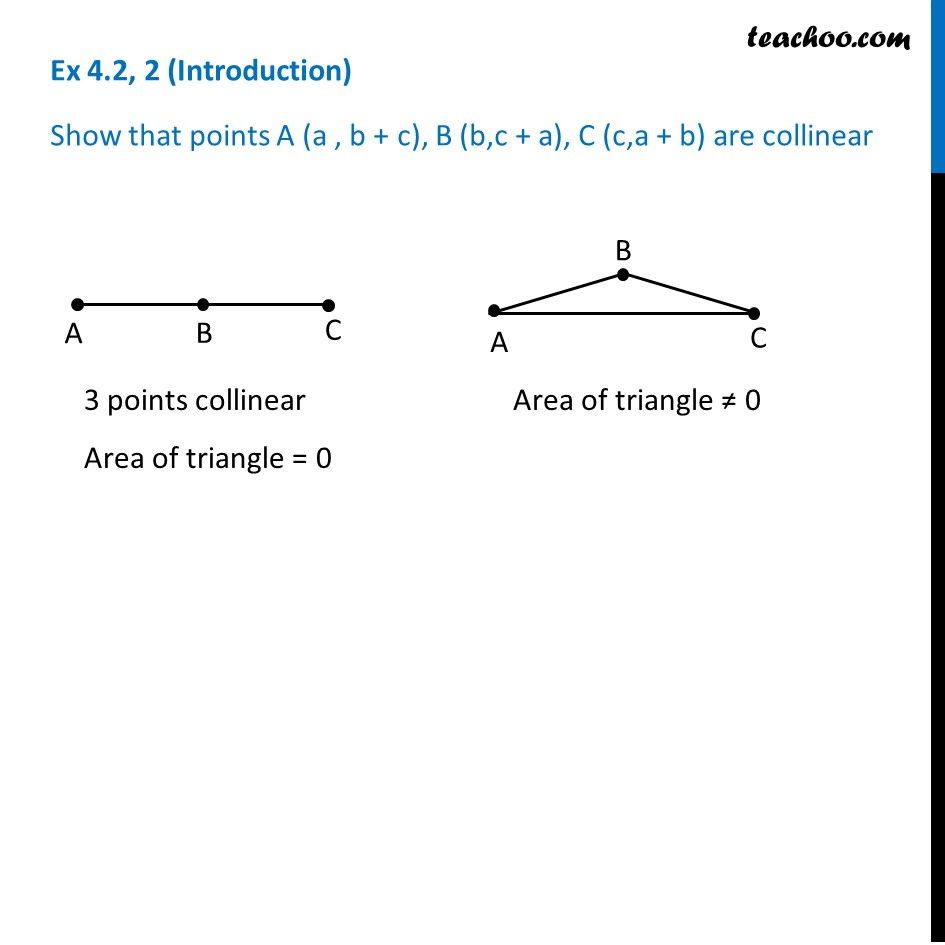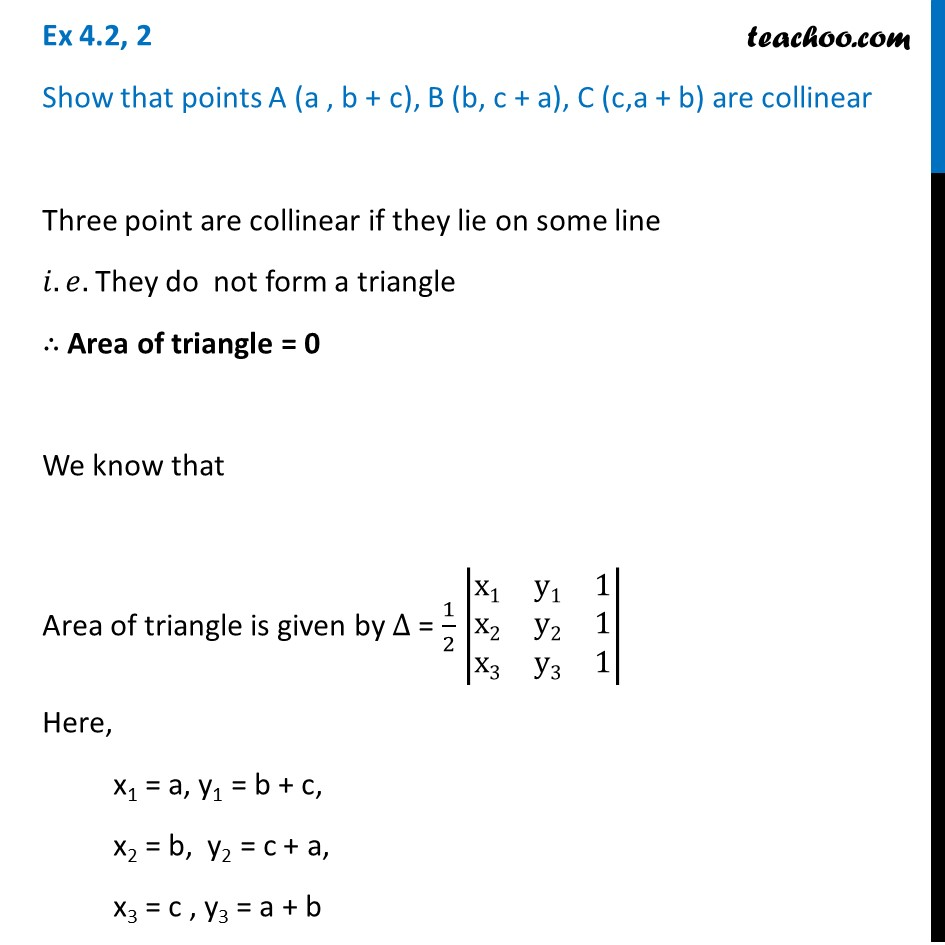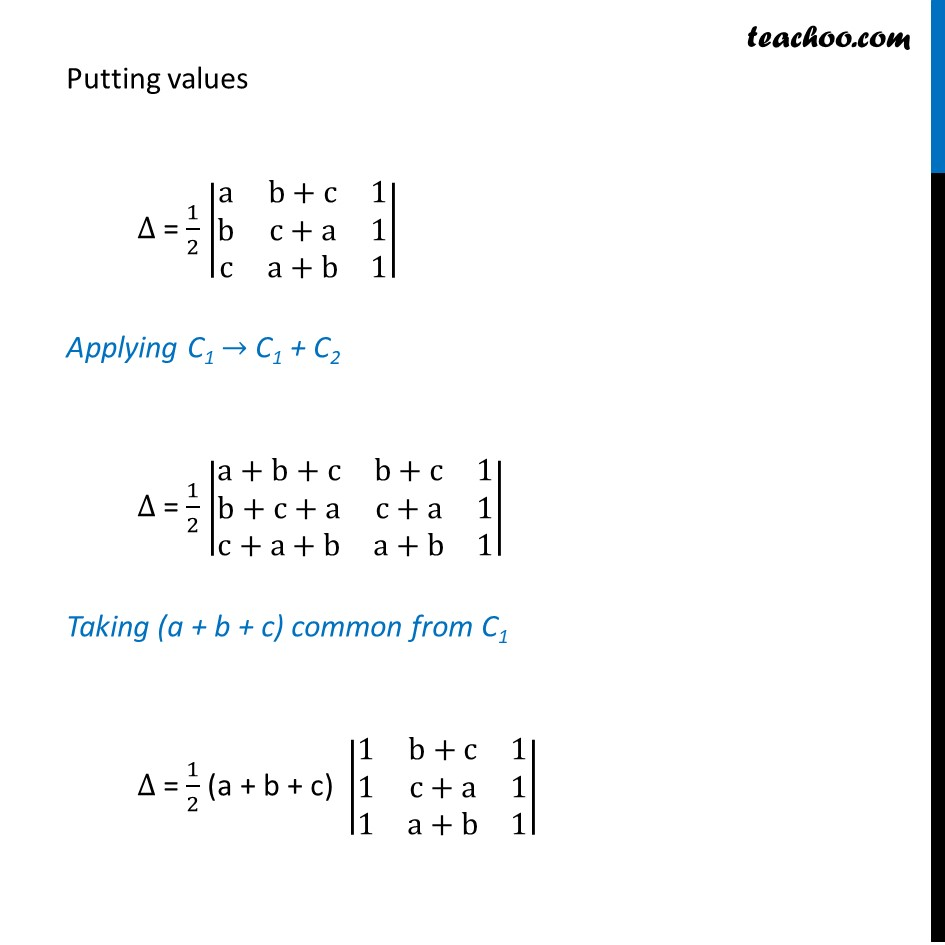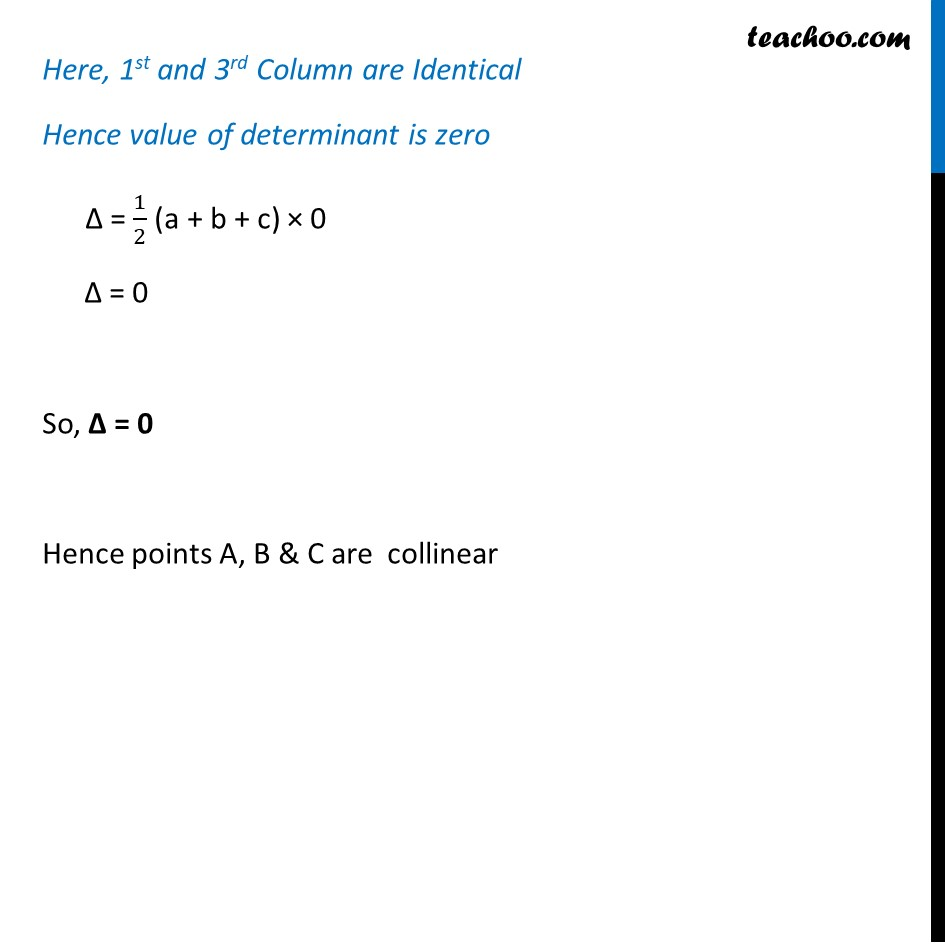Ex 4.2

Chapter 4 Class 12 Determinants
Serial order wiseLearn in your speed, with individual attention - Teachoo Maths 1-on-1 Class

### Transcript

Ex 4.2, 2 (Introduction) Show that points A (a , b + c), B (b,c + a), C (c,a + b) are collinear 3 points collinear Area of triangle = 0 Area of triangle ≠ 0 Ex 4.2, 2 Show that points A (a , b + c), B (b, c + a), C (c,a + b) are collinear Three point are collinear if they lie on some line 𝑖.𝑒. They do not form a triangle ∴ Area of triangle = 0 We know that Area of triangle is given by ∆ = 1/2 |■8(x1&y1&1@x2&y2&1@x3&y3&1)| Here, x1 = a, y1 = b + c, x2 = b, y2 = c + a, x3 = c , y3 = a + b Putting values ∆ = 1/2 |■8(a&b+c&1@b&c+a&1@c&a+b&1)| Applying C1 → C1 + C2 ∆ = 1/2 |■8(a+b+c&b+c&1@b+c+a&c+a&1@c+a+b&a+b&1)| Taking (a + b + c) common from C1 ∆ = 1/2 (a + b + c) |■8(1&b+c&1@1&c+a&1@1&a+b&1)| Here, 1st and 3rd Column are Identical Hence value of determinant is zero ∆ = 1/2 (a + b + c) × 0 ∆ = 0 So, ∆ = 0 Hence points A, B & C are collinear